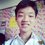# A seemingly hard divisibility problem

For every $n=1,2,3,..., 2040$, prove there exists a multiple of $n$, which has less than $12$ digits, and only contains the digits $0,1,8$ or $9$.

Solution: Take two $11$ digits numbers, consisting of only $0$ and $1$, since there are $2^{11}=2048$ numbers that can be formed, there must be two numbers with the same remainder after dividing by $n$. Thus, subtracting one with another, we get a multiple of $n$ which has less than $12$ digits, and only contains the digits $0,1,8$ or $9$.

Generalization: For every $n=1,2,3,..., k$ where $k \le 2^j-1$, there exists a multiple of $n$, which has less than $j+1$ digits, and only contains the digits $0,1,8$ or $9$.Note by ChengYiin Ong
1 year, 1 month ago

This discussion board is a place to discuss our Daily Challenges and the math and science related to those challenges. Explanations are more than just a solution — they should explain the steps and thinking strategies that you used to obtain the solution. Comments should further the discussion of math and science.

When posting on Brilliant:

• Use the emojis to react to an explanation, whether you're congratulating a job well done , or just really confused .
• Ask specific questions about the challenge or the steps in somebody's explanation. Well-posed questions can add a lot to the discussion, but posting "I don't understand!" doesn't help anyone.
• Try to contribute something new to the discussion, whether it is an extension, generalization or other idea related to the challenge.

MarkdownAppears as
*italics* or _italics_ italics
**bold** or __bold__ bold
- bulleted- list
• bulleted
• list
1. numbered2. list
1. numbered
2. list
Note: you must add a full line of space before and after lists for them to show up correctly
paragraph 1paragraph 2

paragraph 1

paragraph 2

[example link](https://brilliant.org)example link
> This is a quote
This is a quote
    # I indented these lines
# 4 spaces, and now they show
# up as a code block.

print "hello world"
# I indented these lines
# 4 spaces, and now they show
# up as a code block.

print "hello world"
MathAppears as
Remember to wrap math in $$ ... $$ or $ ... $ to ensure proper formatting.
2 \times 3 $2 \times 3$
2^{34} $2^{34}$
a_{i-1} $a_{i-1}$
\frac{2}{3} $\frac{2}{3}$
\sqrt{2} $\sqrt{2}$
\sum_{i=1}^3 $\sum_{i=1}^3$
\sin \theta $\sin \theta$
\boxed{123} $\boxed{123}$

Sort by:

Can you give the numbers? @ChengYiin Ong

- 1 year, 1 month ago

Maybe for $n=11$, i can choose $11100001001$ and $10000000000$, they both have $11$ digits and subtracting the first with the second, we get $1100001001$ which is a multiple of 11 and only contains the digits $0,1,8$ or $9$.

- 1 year, 1 month ago

@ChengYiin Ong - if there are 2048 possibilities then how can you say that "there must be two numbers with the same remainder after dividing by n?"

- 1 year, 1 month ago

there are $2048$ numbers that you can form from choosing only $0$ or $1$ at for every digit and so there must be two numbers that have the same modulo $n$, maybe i shouldn't say "possibility"

- 1 year, 1 month ago